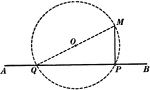### Obtuse Angles Inscribed in Circle Proof

Illustration of a circle used to prove "Any angle inscribed in a segment less than a semicircle is an…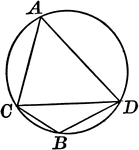### Circle With Inscribed Angle Greater Than Semicircle

Illustration of a circle with an angle inscribed in a segment greater than a semicircle, an acute angle.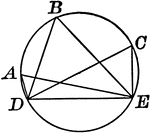### Circle With Inscribed Angle Less Than Semicircle

Illustration of a circle with an angle inscribed in a segment less than a semicircle, an obtuse angle.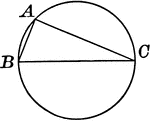### Circle With Inscribed Right Angle in Semicircle

Illustration of a circle with a right angle inscribed in a semicircle.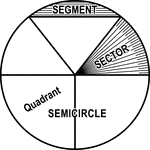### Circle with Segment, Semicircle, Sector, Quadrant, and Central Angle

Illustrations of a circle with segment, semicircle, sector, quadrant, and central angles drawn.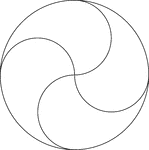### Circular Design

Circular design made by rotating circles about a fixed point. The radii of the smaller circles is equal…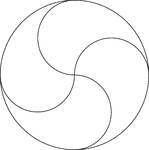### Circular Design

Circular design made by rotating circles about a fixed point. The radii of the smaller circles is equal…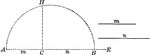### Semicircle

Semicircle with line drawn perpendicular to the diameter.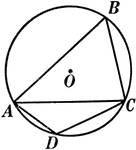### Right Angles Inscribed in Semicircle Proof

Illustration of a circle used to prove "Any angle inscribed in a semicircle is a right angle."### Semicircle With Chords and Radii

Illustration of a semicircle with chords and radii.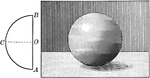### Sphere Made by Revolution of Semicircle

Illustration of a sphere generated by the revolution of a semicircle ACB about its diameter AB as an…